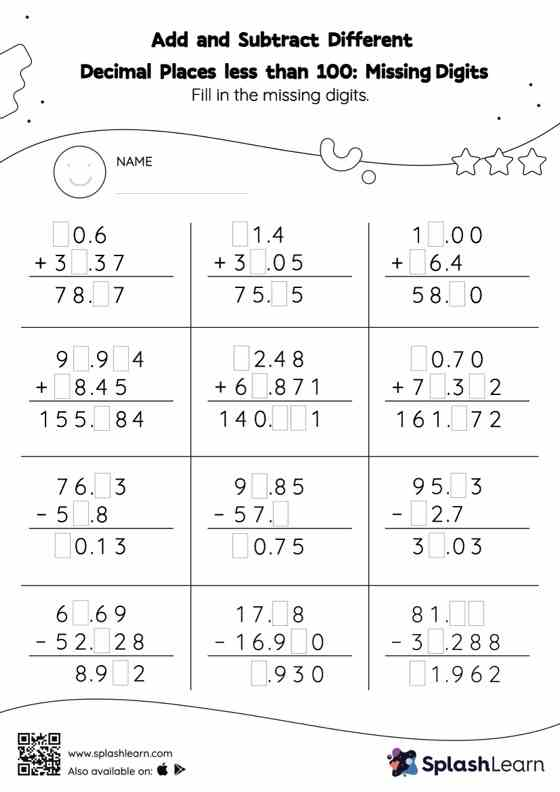# Add and Subtract Different Decimal Places less than 100: Missing Digits Worksheet

Home > Add and Subtract Different Decimal Places less than 100: Missing DigitsDoes your child know how to add and subtract different decimal places less than 100? Invite them to practice this concept here. In this worksheet, students add and subtract decimals by aligning the decimal points and using zero as a placeholder. They then use the relationship between addition and subtraction to find the missing number in the add and subtract different decimal places less than 100 worksheet.